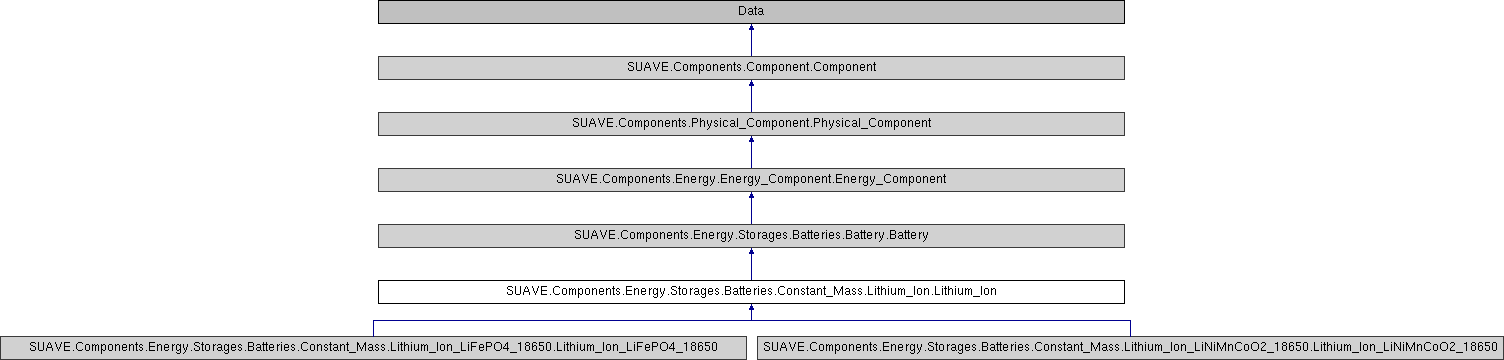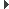SUAVE  2.5.2 An Aerospace Vehicle Environment for Designing Future AircraftSUAVE.Components.Energy.Storages.Batteries.Constant_Mass.Lithium_Ion.Lithium_Ion Class Reference
Inheritance diagram for SUAVE.Components.Energy.Storages.Batteries.Constant_Mass.Lithium_Ion.Lithium_Ion:## Public Member Functions

def __defaults__ (self)

def energy_calc (self, numerics, battery_discharge_flag=True)

def append_battery_unknowns (self, segment)

def append_battery_residuals (self, segment, network)

def append_battery_unknowns_and_residuals_to_segment (self, segment, initial_voltage, initial_battery_cell_temperature, initial_battery_state_of_charge, initial_battery_cell_current)

def compute_voltage (self, state)

def update_battery_state_of_health (self, segment, increment_battery_cycle_day=False)

## Public Attributes

tag

cell

module

pack_config

module_config

age

convective_heat_transfer_coefficient

heat_transfer_efficiency

cooling_fluid

specific_energy

specific_powerPublic Attributes inherited from SUAVE.Components.Energy.Storages.Batteries.Battery.Battery
chemistry

energy_density

current_energy

initial_temperature

current_capacitor_charge

resistance

specific_heat_capacity

max_energy

max_power

max_voltage

discharge_performance_map

ragonePublic Attributes inherited from SUAVE.Components.Energy.Energy_Component.Energy_Component
inputs

outputsPublic Attributes inherited from SUAVE.Components.Physical_Component.Physical_Component
tag

mass_properties

origin

symmetricPublic Attributes inherited from SUAVE.Components.Component.Component
tag

origin

generative_design_max_per_vehicle

generative_design_characteristics

generative_design_special_parent

## Detailed Description

```Generic lithium ion battery that specifies discharge/specific energy
characteristics.

Assumptions
1) Default discharge curves correspond to lithium-iron-phosphate cells

2) Convective Thermal Conductivity Coefficient corresponds to forced
air cooling in 35 m/s air

Inputs:
None

Outputs:
None

Properties Used:
N/A
```

## ◆ __defaults__()

 def SUAVE.Components.Energy.Storages.Batteries.Constant_Mass.Lithium_Ion.Lithium_Ion.__defaults__ ( self )
```This sets the default inputs and outputs data structure.

Assumptions:
None

Source:
N/A

Inputs:
None

Outputs:
None

Properties Used:
N/A
```

Reimplemented from SUAVE.Components.Energy.Storages.Batteries.Battery.Battery.

## ◆ append_battery_residuals()

 def SUAVE.Components.Energy.Storages.Batteries.Constant_Mass.Lithium_Ion.Lithium_Ion.append_battery_residuals ( self, segment, network )
```Packs the residuals specific to LFP cells to be sent to the mission solver.

Assumptions:
None

Source:
N/A

Inputs:
state.conditions.propulsion:
motor_torque                          [N-m]
propeller_torque                      [N-m]

Outputs:
None

Properties Used:
network.voltage                           [volts]
```

## ◆ append_battery_unknowns()

 def SUAVE.Components.Energy.Storages.Batteries.Constant_Mass.Lithium_Ion.Lithium_Ion.append_battery_unknowns ( self, segment )
```Appends unknowns specific to LFP cells which are unpacked from the mission solver and send to the network.

Assumptions:
None

Source:
N/A

Inputs:

Outputs:

Properties Used:
N/A
```

## ◆ append_battery_unknowns_and_residuals_to_segment()

 def SUAVE.Components.Energy.Storages.Batteries.Constant_Mass.Lithium_Ion.Lithium_Ion.append_battery_unknowns_and_residuals_to_segment ( self, segment, initial_voltage, initial_battery_cell_temperature, initial_battery_state_of_charge, initial_battery_cell_current )
```Sets up the information that the mission needs to run a mission segment using this network

Assumptions:
None

Source:
N/A

Inputs:
initial_voltage                       [volts]
initial_battery_cell_temperature      [Kelvin]
initial_battery_state_of_charge       [unitless]
initial_battery_cell_current          [Amperes]

Outputs
None

Properties Used:
N/A
```

## ◆ compute_voltage()

 def SUAVE.Components.Energy.Storages.Batteries.Constant_Mass.Lithium_Ion.Lithium_Ion.compute_voltage ( self, state )
```Computes the voltage of a single LFP cell or a battery pack of LFP cells

Assumptions:
None

Source:
N/A

Inputs:
state   - segment unknowns to define voltage [unitless]

Outputs

Properties Used:
N/A
```

## ◆ energy_calc()

 def SUAVE.Components.Energy.Storages.Batteries.Constant_Mass.Lithium_Ion.Lithium_Ion.energy_calc ( self, numerics, battery_discharge_flag = `True` )
```This is an electric cycle model for 18650 lithium-iron_phosphate battery cells. It
models losses based on an empirical correlation Based on method taken
from Datta and Johnson.

Assumptions:
1) Constant Peukart coefficient
2) All battery modules exhibit the same themal behaviour.

Source:
Internal Resistance:
Nikolian, Alexandros, et al. "Complete cell-level lithium-ion electrical ECM model
for different chemistries (NMC, LFP, LTO) and temperatures (− 5° C to 45° C)–
Optimized modelling techniques." International Journal of Electrical Power &
Energy Systems 98 (2018): 133-146.

Voltage:
Chen, M. and Rincon-Mora, G. A., "Accurate Electrical
Battery Model Capable of Predicting Runtime and I - V Performance" IEEE
Transactions on Energy Conversion, Vol. 21, No. 2, June 2006, pp. 504-511

Inputs:
battery.
I_bat             (max_energy)                          [Joules]
cell_mass         (battery cell mass)                   [kilograms]
Cp                (battery cell specific heat capacity) [J/(K kg)]
E_max             (max energy)                          [Joules]
E_current         (current energy)                      [Joules]
Q_prior           (charge throughput)                   [Amp-hrs]
R_growth_factor   (internal resistance growth factor)   [unitless]
E_growth_factor   (capactance (energy) growth factor)   [unitless]

inputs.
I_bat             (current)                             [amps]
P_bat             (power)                               [Watts]

Outputs:
battery.
current_energy                                           [Joules]
cell_temperature                                         [Kelvin]
resistive_losses                                         [Watts]